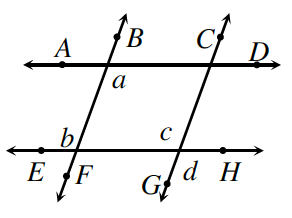### Home > CCG > Chapter 7 > Lesson 7.3.1 > Problem7-124

7-124.Carolina compared her proof to Penn’s work in problem 7-113. Like him, she wanted to prove that if $\overline{AD}//\overline{EH}$ and $\overline{BF}//\overline{CG}$, then $a=d$. Unfortunately, her statements were in a different order. Examine her proof below and help her decide if her statements are in a logical order in order to prove that $a=d$.

Statements

Reasons

1. $\overline{AD}//\overline{EH}$ and $\overline{BF}//\overline{CG}$

• Given

1. $a=b$

• If lines are parallel, alternate interior angles are equal.

1. $a=c$

• Substitution

1. $b=c$

• If lines are parallel, corresponding angles are equal.

1. $c=d$

• Vertical angles are equal.

1. $a=d$

• SubstitutionIs it possible to know that $a=c$ in the 3rd statement? What step is out of order?